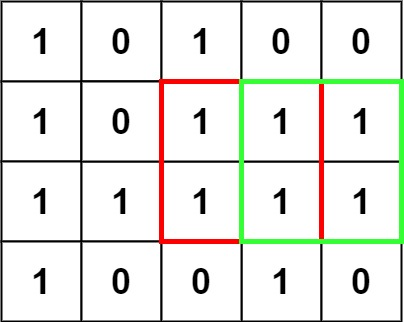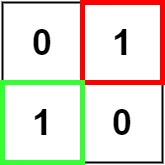## 221. Maximal Square

Given an `m x n` binary `matrix` filled with `0`'s and `1`'s, find the largest square containing only `1`'s and return its area.

Example 1:```Input: matrix = [["1","0","1","0","0"],["1","0","1","1","1"],["1","1","1","1","1"],["1","0","0","1","0"]]
Output: 4
```

Example 2:```Input: matrix = [["0","1"],["1","0"]]
Output: 1
```

Example 3:

```Input: matrix = [["0"]]
Output: 0
```

Constraints:

• `m == matrix.length`
• `n == matrix[i].length`
• `1 <= m, n <= 300`
• `matrix[i][j]` is `'0'` or `'1'`.

## Rust Solution

``````struct Solution;

impl Solution {
fn maximal_square(matrix: Vec<Vec<char>>) -> i32 {
let mut max = 0;
let n = matrix.len();
if n == 0 {
return 0;
}
let m = matrix.len();
if m == 0 {
return 0;
}
let mut dp: Vec<Vec<i32>> = vec![vec![0; m + 1]; n + 1];
for i in 1..=n {
for j in 1..=m {
if matrix[i - 1][j - 1] == '1' {
dp[i][j] = i32::min(dp[i - 1][j - 1], i32::min(dp[i - 1][j], dp[i][j - 1])) + 1;
max = i32::max(max, dp[i][j]);
}
}
}
max * max
}
}

#[test]
fn test() {
let matrix = vec_vec_char![
['1', '0', '1', '0', '0'],
['1', '0', '1', '1', '1'],
['1', '1', '1', '1', '1'],
['1', '0', '0', '1', '0']
];
assert_eq!(Solution::maximal_square(matrix), 4);
}
``````

Having problems with this solution? Click here to submit an issue on github.